Next: Solutions and Distinct solutions Up: Mathematical background Previous: Managing the bisection and   Contents

An alternative: the single bisection

A possibility to reduce the combinatorial explosion of the previous algorithm is to bisect not all the variables i.e. to use the full bisection mode, but only one of them (it must be noted that the algorithms in ALIAS will not accept a full bisection mode if the number of unknowns exceed 10). This may reduce the computation time as the number of function evaluation may be reduced. But the problem is to determine which variable should be bisected. All the solving algorithms of ALIAS may manage this single bisection by setting the flag Single_Bisection to a value different from 0. The value of this global variable indicates various bisection modes. Although the behavior of the mode may change according to the algorithm here are the possible modes for the general solving algorithm and the corresponding values for Single_Bisection:
• 1 : we just split the variable having the largest width (valid for all algorithms). Note however that it is still possible to order the bisection i.e. to split first a subset of the unknowns until their width is small (i.e. lower than ALIAS_Accuracy, then another subset and so on. This is obtained by setting flag ALIAS_Ordered_Bisection to 1 and defining an integer matrix ALIAS_Order_Bisection whose rows indicate the bisected subset and should end by 0. For example if this matrix has as rows [1,3,0],[2,4,5,0], then the algorithm will first bisect the unknowns 1 and 3 until their width is small, then the unknowns 2,4,5. If all unknowns indicated in the rows of the matrix have a small width, then the bisection algorithm revert to the normal behavior.
• 2: to determine the variable that will be bisected we use the following approach: we compute the order criteria for the two boxes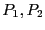that will result from the bisection of variable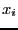and retain the lowest criteria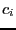. The variable that will be bisected is the one that has the lowestexcept if for at least one variable the interval evaluation of the function for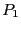or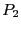does not contain 0. In that case the variable that will be bisected is the one that verify the previous property and which has the lowestamong all the input intervals having the property. However to avoid bisecting over and over the same variable we use another test: let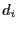be the width of the interval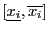and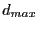be the maximum of all the. If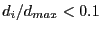we don't consider the variableas a possible bisection direction. It is also possible to mix this mode with mode 1. If the integer variable ALIAS_RANDG is set to a strictly positive value then ALIAS_RANDG bisection will be performed using mode 2 while the next bisection will be performed using mode 1 and the process will be repeated
• 3, 4 : similar to 1
• 5 : we use a round-robin mode i.e. each variable is bisected in turn (first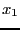, then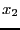and so on) unless the width of the input intervals is less than the desired accuracy on the variable, in which case the bisected variable is the next one having a sufficient width (valid for all algorithms) The flag ALIAS_Round_Robin is used to indicate at each bisection which variable should be bisected.
• 6: we emulate the smear function (see section 2.4.1.3) with an estimation of the gradient based on finite difference (procedure Select_Best_Direction_Grad)
• 7: here again we use the flag ALIAS_Ordered_Bisection set to 1 and defining an integer matrix ALIAS_Order_Bisection whose rows indicates an order for bisecting the unknowns. The largest variable in the first row will be bisected first and so on until all the variables in a row have a width lower than ALIAS_Accuracy. We then proceed to the second row. As soon as all variables in all rows have a width lower than ALIAS_Accuracy we use the bisection 1.
• 20: the user has defined its own bisection procedure, see section 11.3
For all general purpose solving procedures the number of the variable that has been bisected is available in the integer ALIAS_Selected_For_Bisection.

There is another mode called the mixed bisection: among thevariables we will bisect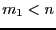variables, which will lead to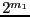new boxes. This mode is obtained by setting the global integer variable
ALIAS_Mixed_Bisection to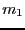. Whatever is the value of Single_Bisection we will order the variables according to their width and select thevariables having the largest width.Next: Solutions and Distinct solutions Up: Mathematical background Previous: Managing the bisection and   Contents
Jean-Pierre Merlet 2012-12-20